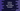# Example to use Numpy log2 method to find natural logarithm of an array of numbers## Example to use Numpy log2 method to find natural logarithm of an array of numbers:

log2 method of Numpy is used to find out the base-2 logarithm of an array of numbers. This method is defined as below:

``numpy.log2(x, /, out=None, *, where=True, casting='same_kind', order='K', dtype=None, subok=True[, signature, extobj]) = <ufunc 'log2'>``

Here,

• x is the input array_like values passed to this method as input.
• out is the location to put the output array. It is an optional value. If not provided, it will put the output in a freshly allocated array. Else, we must provide one array of similar shape to the input array.
• where is a condition. This condition is broadcasted over the input array. Whereever it is True, the output array will be set to the ufunc result. Else, it is remain same.
• **kwargs is for other keyword only arguments.

### Example of log2:

Let’s take a look at the below function:

``````import numpy as np

given_array = [1, 10, 100, 3**9, 98]
print("Given array :",given_array)

new_array = np.log2(given_array)
print("log2 array :",new_array)``````

It will print the below output:

``````Given array : [1, 10, 100, 19683, 98]
log2 array : [ 0.          3.32192809  6.64385619 14.26466251  6.61470984]``````

official doc